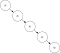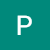# Line by line level order traversal of a binary tree and its related problems

In this article , let us discuss how we can write code for line by line level order traversal. For the above figure, line by line level order traversal would be:
1//1st level
2 3//2nd level
5 6//3rd level

Java Code:

public static void printLevel(Node root){
if(root==null)return;
Queue<Node> q=new LinkedList<>();
q.add(root);
while(q.isEmpty()==false){
int count=q.size();
for(int i=0;i<count;i++){
Node curr=q.poll();
System.out.print(curr.key+” “);
if(curr.left!=null)
q.add(curr.left);
if(curr.right!=null)
q.add(curr.right);
}
System.out.println();
}
}

Approach:

We use two loops, one while loop(outer loop) and one for loop(inner loop). Outer loop is used to stop the processing after each element is printed.Inner loop is used to print…

# Self balancing binary search trees

What is is a self balancing binary search tree and how it is different from normal Binary Search Tree(BST):

Normal BST can be skewed(all nodes will be either on the left or right side) or height difference between left and right sub tree is large, if you want to do any operation on normal BST , you would have to traverse whole tree in worst case. It would be of time complexity O(n)(analogous to operations on LinkedList).Unbalanced binary search tree.

This is where self balancing binary search trees come to the rescue, they adjust the height such that typically height will be maintained…## Kashyap Palle

Software Engineer interested in designing scalable distributed systems.